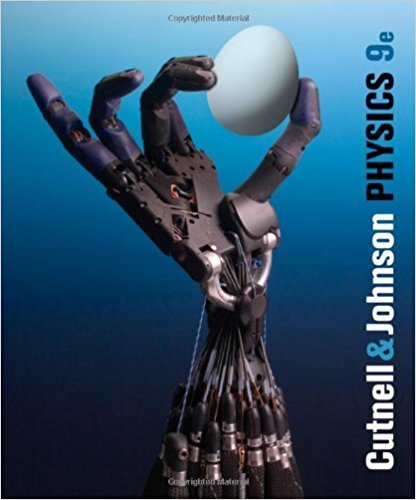×
Log in to StudySoup
Get Full Access to Physics, - 9 Edition - Chapter 23 - Problem 23.2
Join StudySoup for FREE
Get Full Access to Physics, - 9 Edition - Chapter 23 - Problem 23.2

Already have an account? Login here
×
Reset your password

# One circuit contains only an ac generator and a resistor,ISBN: 9780470879528 211

## Solution for problem 23.2 Chapter 23

Physics, | 9th Edition

• Textbook Solutions
• 2901 Step-by-step solutions solved by professors and subject experts
• Get 24/7 help from StudySoup virtual teaching assistantsPhysics, | 9th Edition

4 5 1 346 Reviews
31
5
Problem 23.2

One circuit contains only an ac generator and a resistor, and the rms current in this circuit is IR. Another circuit contains only an ac generator and a capacitor, and the rms current in this circuit is IC. The maximum, or peak, voltage of the generator is the same in both circuits and does not change. If the frequency of each generator is tripled, by what factor does the ratio IR/IC change? Specify whether the change is an increase or a decrease.

Step-by-Step Solution:
Step 1 of 3

Lecture 7 Potential from a distribution of charges X V = 1 qi 4⇡✏0 ri §Smooth distribution 1 X qi 1 Z ⇢ V = = dV 4⇡✏0 i ri 4⇡✏ 0 r § of point charges is usually much simpler thanup calculating the electric field •It’s a scalar Electric Potential from Two Oppositely Charged Point Charges §The electric field lines from two oppositely charge point charges are a little more complicated §The electric field lines originate on the positive charge and terminate on the negative charge §The equipotential lines are always perpendicular to the electric field lines §The red lines represent positive electric potential §The blue lines represent n

Step 2 of 3

Step 3 of 3

##### ISBN: 9780470879528

This full solution covers the following key subjects: . This expansive textbook survival guide covers 32 chapters, and 3930 solutions. Since the solution to 23.2 from 23 chapter was answered, more than 279 students have viewed the full step-by-step answer. This textbook survival guide was created for the textbook: Physics,, edition: 9. Physics, was written by and is associated to the ISBN: 9780470879528. The full step-by-step solution to problem: 23.2 from chapter: 23 was answered by , our top Physics solution expert on 01/04/18, 08:42PM. The answer to “One circuit contains only an ac generator and a resistor, and the rms current in this circuit is IR. Another circuit contains only an ac generator and a capacitor, and the rms current in this circuit is IC. The maximum, or peak, voltage of the generator is the same in both circuits and does not change. If the frequency of each generator is tripled, by what factor does the ratio IR/IC change? Specify whether the change is an increase or a decrease.” is broken down into a number of easy to follow steps, and 82 words.

Unlock Textbook Solution

Enter your email below to unlock your verified solution to:

One circuit contains only an ac generator and a resistor,